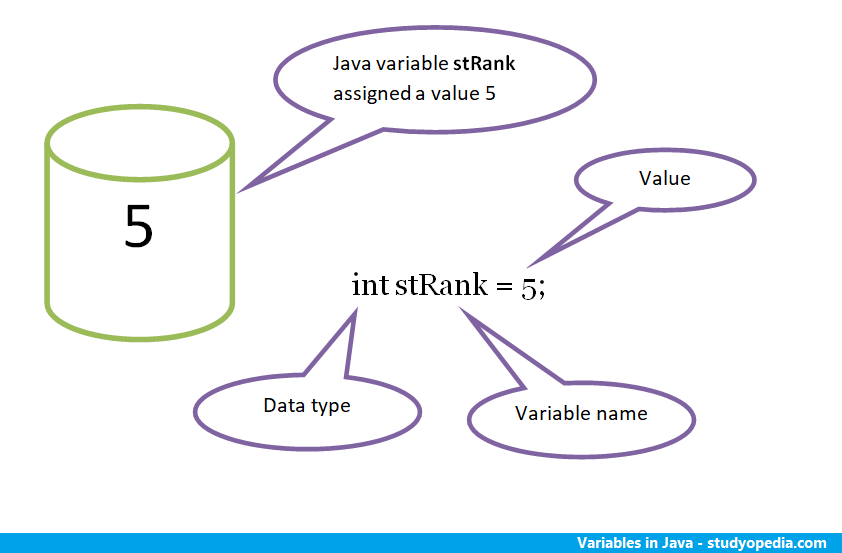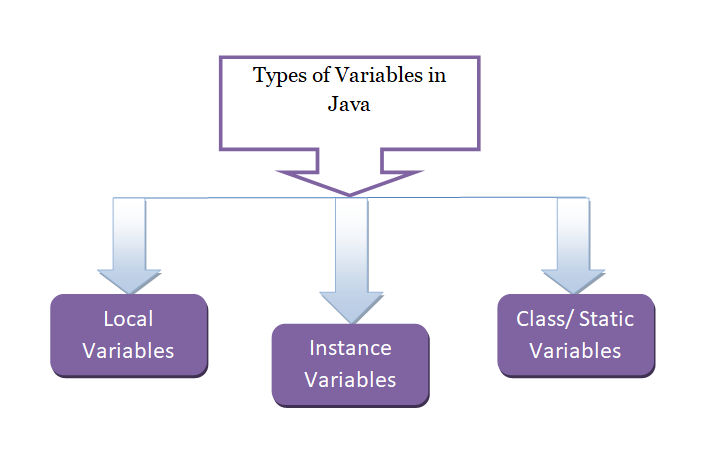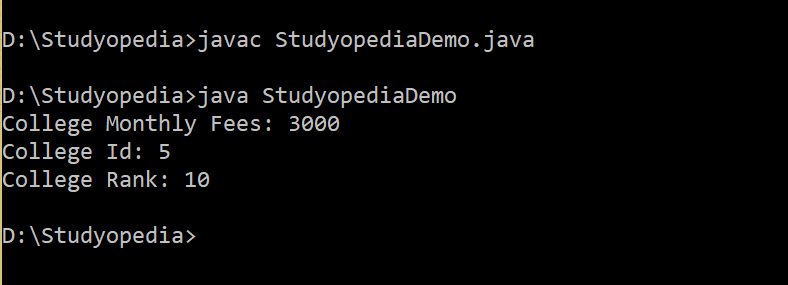# Java Variables

## 24 Aug Java Variables

In this lesson, we will learn how to work with Java Variables. Variables in Java are a reserved area allocated in memory. It is a container which holds value. Each variable is assigned a type, which is known as data type. We will also work around the types of  Java variables, including Local, Instance and Class Variable.

The reserved area set for the variable stRank as shown below. Here, the variable is assigned a value 5. Also, you can see, we need a datatype to declare a variable. The int data type is used below.Declare Variables

As in the above figure, variables are declared by adding a data type, with the variable name.

Here are some examples:

Initialize Variables

To initialize a variable, assign a value to it. Here are some examples:

Here’s the usage of variable in a Java program:

For example,

The value of the integer variable above is assigned 90.

Here are the types of variables in Java:## Local Variable

A local variable is assigned a value locally. It is a variable that gets declared inside a method and can be accessed inside the same method only.

For example, int rank = 10;

Let’s see the complete example,

## Instance Variable

A variable which is declared in a class, but outside the method, is known as instance variable. It is not declared as static.

For example, int id =5;

Let’s see the complete example,

## Class/ Static Variable

A class variable is declared static. Class variable is also known as static variable.

For example, static int fees;

Let’s see the complete example,

Here’s the output for the above program, which shows the usage of Java variables.

Run the above program in the same way and you will get the following output:##### Tags:[email protected]

We work to create free tutorials for all.